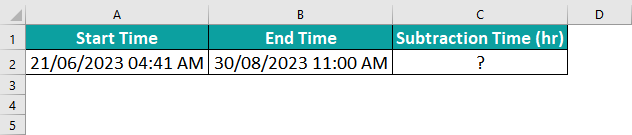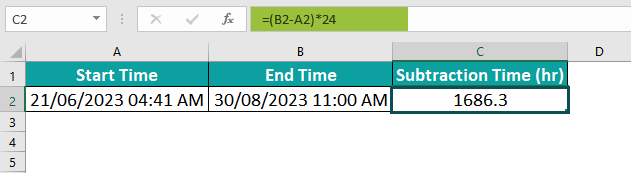# Subtract Time In ExcelArticle byExcelMojo Team## What Is Subtract Time In Excel?

Subtracting time in Excel refers to calculating the difference between two given time values. This functionality is essential for various professional applications, such as calculating working hours, determining project durations, or measuring response times. When subtracting time in Excel, one must remember the underlying format and precision of the time values used. Excel stores time as decimal fractions, with 1 representing a full day (24 hours). To perform a subtraction, subtract a one-time value from another using the appropriate syntax or formula. It’s important to correctly format and display the result by utilizing formatting options provided by Excel to represent it as a recognizable time duration.

In the following, we are provided with the start and finish times, allowing us to determine the total time taken. These time values in columns A and B are recorded, respectively.

To find the duration in hours, we subtract the start time from the finish time using the “-” operator. Now, we have to multiply the value by 24.

The formula employed for this calculation is =(B2-A2)*24. This process is straightforward as the time difference always falls within 24 hours.

In this particular instance, the result is 9.5 hours.

###### Key Takeaways
• Subtract time in Excel is a function that finds the difference between two given time values.
• To obtain the total number of hours after subtracting time values, we can use the ‘INT’ function and round it to the nearest whole number. This ensures that we have an accurate representation of the hours involved.
• It is worth mentioning that if a cell, after applying custom formatting, displays a sequence of ‘#####,’ it is likely due to the cell’s width being insufficient to display the date-time value properly. Adjusting the cell width will resolve this issue and allow for the correct value display.

### How To Subtract Time In Excel? (With Steps)

• First, ensure the cells containing our time values are properly formatted as time.
• Select the cell where we want the difference to appear and enter the subtraction formula: “=end_time – start_time.”
• Replace “end_time” with the excel cell reference of the later time and “start_time” with the cell reference of the earlier time.
• Press Enter to calculate the difference. To display it in a more readable format, right-click on the cell, select Format Cells in excel, go to the Number tab, click Time under Category, choose a suitable format from the list or specify our custom style using h:mm: ss format code.
• Additionally, to account for negative times resulting from crossing midnight boundaries, apply a conditional formatting rule using formulas using built-in time functions such as IF and ABS.
• Extracting time in Excel allows us to efficiently perform calculations and analyze data involving durations or elapsed times precisely and accurately.

### Examples

#### Example #1

In this example, we are provided with the start time and finish time of a test completed by some students, and we can find the total time taken by these students to complete the test.

Step 1: The start and finish times are recorded in columns B and C, respectively.

Step 2: To find the total time taken in hours, we subtract the start time from the finish time using the “-” operator. We then multiply the resulting value by 24.

The formula used is =(C2-B2)*24.

Step 3: This calculation is straightforward since the time difference is always within 24 hours. In this case, the result is 2.5 hours.

Step 4: To apply this formula to multiple rows, drag the formula from cell D2 to D6.

#### Example #2

Now, let’s consider a scenario where we need to subtract the time in Excel that exceeds 24 hours when the start and finish times are provided.

Step 1: In this case, the Start and Finish times are recorded in columns A and B, respectively. We aim to calculate the total time taken (in hours) and display it in column C.

Step 2: We can subtract the start time from the finish time using the “-” operator to achieve this. To obtain the total time taken in hours, we have to multiply the resulting value by 24.

The formula used is =(B2-A2)*24.

Step 3: If we apply this calculation to the given data, the total time is 25.0 hours.

Step 4: To apply this formula to multiple rows, we can drag the formula from cell C2 to C7.

It is worth noting that these Date Time values span across midnight and are represented using custom formats. These formats allow us to display time intervals that exceed the standard time units’ length.

#### Example #3

Let us consider the below example where we must subtract a specific time interval from a given time.

Step 1: This can be accomplished by dividing the number of hours, minutes, or seconds by the corresponding unit in a day (24 hours, 1440 minutes, 86400 seconds) and subtracting the resulting quotient from the given time.

The formula used is =A2-(B2/24).

Step 2: When the time to be subtracted is less than 24 hours, we can observe that the Given Time is located in column A, while column B contains the number of hours we intend to subtract. The subtraction of these two-time values can be performed as follows:

Time Taken = Given Time – (Number of hours to be subtracted / 24)

Step 3: If we apply this calculation to the given data, the result is 17:00:00.

Step 4: To apply this formula to multiple rows, we can drag the formula from cell C2 to C6.

### Important Things To Note

• In Excel, we can add hours, minutes, and seconds to a Date Time using custom number formats. These formats allow us to properly display time values that exceed the standard 24 hours, 60 minutes, or 60 seconds.
• It is important to note that custom time formats are designed to work with positive time values. This means negative time values will not be properly formatted using these custom formats.
• In cases where the Finish Time exceeds the Start Time, the resulting time difference will be shown as a negative number. This allows us to easily identify situations where the finish time occurs after the start time.

1. Are there any limitation when subtracting time in Excel?

• Excel stores times as decimal values, where one day equals 1. Therefore, when subtracting time, the result is displayed as a decimal value representing the number of days.
• Further calculations or formatting adjustments may be necessary to convert this value into a recognizable time format (such as hours or minutes).

2. Are there any common mistakes or errors to be aware of when subtracting time in Excel?

• Excel stores time as a decimal value, with one day as 1. Therefore, if we want to subtract two-time values accurately, we must first convert them to decimals by dividing them by 24 (since there are 24 hours a day) and then performing the subtraction.
• Another mistake to avoid is subtracting time values without considering the date component. Excel treats date and times as separate entities. So, we have to ensure the corresponding dates are properly considered. This is to avoid incorrect results when subtracting time across days or months.

3. How can I customize the display format for the result of a time subtraction in Excel?

In the following section, we are presented with the start and finish times, enabling us to calculate the total time taken accurately. These time values are conveniently recorded in columns A and B, respectively.To determine the duration in hours, we subtract the start time from the finish time using the “-” operator. The resulting value is then multiplied by 24.

The formula utilized for this calculation is =(B2-A2)*24. This process is remarkably straightforward, especially when the time difference exceeds 24 hours.

In this specific case, the outcome is a duration of 1686.3 hours.